来自现实世界的邀请：映射转换 | 带你学《Java面向对象编程》之二十三

+关注继续查看

【本节目标】

数据表与简单Java类映射转换

• 数据实体表设计 = 类的定义；
• 表中的字段 = 类的成员属性；
• 表的外键关联 = 引用关联；
• 表的一行记录 = 类的一个实例化对象；
• 表的多行记录 = 对象数组。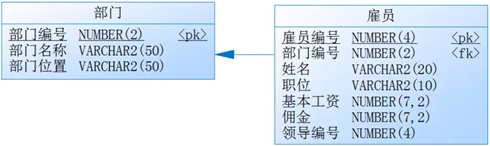• 一个部门有多个雇员；
• 一个雇员属于一个部门；
• 一个雇员有一个领导；

• 根据部门信息获得以下内容：

• 一个部门的完整信息；
• 一个部门之中所有雇员的完整信息；
• 一个雇员对应的领导的信息；
• 根据雇员信息获得以下内容：

• 一个雇员对应的所在部门信息；
• 一个雇员对应的领导信息；

class Dept {
private long deptno ;
private String dname ;
private String loc ;
private Emp emps [] ;          //多个雇员信息
public Dept(long deptno ,String dname ,String loc) {
this.deptno = deptno ;
this.dname = dname ;
this.loc = loc ;
}
public void setEmps(Emp [] emps) {
this.emps = emps ;
}
public Emp [] getEmps () {
return this.emps ;
}
//setter、getter、无参构造略
public String getInfo() {
return “【部门信息】部门编号 = ” + this.deptno + “、部门名称 = ” + this.dname + “、部门位置 = ” + this.loc ;
}
}
class Emp {
private long empno ;
private String ename ;
private String job ;
private double sal ;
private double comm ;
private Dept dept ;       //所属部门
private Emp mgr ;        //所属领导
public Emp(long empno ,String ename ,String job ,double sal ,double comm) {
this.empno = empno ;
this.ename =ename ;
this.job = job ;
this.sal = sal ;
this.comm = comm ;
}
//setter、getter、无参构造略
public String getInfo() {
return “【雇员信息】雇员编号 = ” + this.empnno + “、雇员姓名 = ” + this.ename + “、雇员职位 = ” + this.job + “、基本工资 = ” + this.sal + “、佣金 = ” + this.comm ;
}
public void setDept(Dept dept) {
this.dept = dept ;
}
public void setMgr(Emp mgr) {
this.mgr= mgr ;
}
public Dept getDept() {
return this.dept ;
}
public Emp getMgr() {
return this.mgr ;
}
}
public static void main(String args[]){

}
}

• 第一步：根据表的结构关系进行对象的配置；
• 第二步：根据要求通过结构获取数据。

class Dept {
private long deptno ;
private String dname ;
private String loc ;
private Emp emps [] ;          //多个雇员信息
public Dept(long deptno ,String dname ,String loc) {
this.deptno = deptno ;
this.dname = dname ;
this.loc = loc ;
}
public void setEmps(Emp [] emps) {
this.emps = emps ;
}
public Emp [] getEmps () {
return this.emps ;
}
//setter、getter、无参构造略
public String getInfo() {
return “【部门信息】部门编号 = ” + this.deptno + “、部门名称 = ” + this.dname + “、部门位置 = ” + this.loc ;
}
}
class Emp {
private long empno ;
private String ename ;
private String job ;
private double sal ;
private double comm ;
private Dept dept ;       //所属部门
private Emp mgr ;        //所属领导
public Emp(long empno ,String ename ,String job ,double sal ,double comm) {
this.empno = empno ;
this.ename =ename ;
this.job = job ;
this.sal = sal ;
this.comm = comm ;
}
//setter、getter、无参构造略
public String getInfo() {
return “【雇员信息】雇员编号 = ” + this.empnno + “、雇员姓名 = ” + this.ename + “、雇员职位 = ” + this.job + “、基本工资 = ” + this.sal + “、佣金 = ” + this.comm ;
}
public void setDept(Dept dept) {
this.dept = dept ;
}
public void setMgr(Emp mgr) {
this.mgr= mgr ;
}
public Dept getDept() {
return this.dept ;
}
public Emp getMgr() {
return this.mgr ;
}
}
public static void main(String args[]){
//第一步：根据关系进行类的定义
//定义出各个的实例对象，此时并没有任何的关联定义
Dept dept = new Dept(10 ,”财务部” ,”上海”) ;
Emp empA = new Emp(7369L ,”SMITH” ,”CLERK” ,800.00 ,0.0) ;
Emp empB= new Emp(7566L ,”FORD” ,”MANAGER” ,2450.00 ,0.0) ;
Emp empC = new Emp(7839L ,”KING” ,”PRESIDENT” ,5000.00 ,0.0) ;
//需要为对象进行关联的设置
empA.setDept(dept) ;    //设置雇员与部门之间的关联
empB.setDept(dept) ;    //设置雇员与部门之间的关联
empC.setDept(dept) ;    //设置雇员与部门之间的关联
empA.setMgr(empB) ;    //设置雇员与领导的关联
empB.setMgr(empC) ;    //设置雇员与领导的关联
dept.setEmps(new Emp[ ] {empA ,empB ,empC}) ;  //部门与雇员的关联
//第二步：根据关系获取数据
System.out.println(dept.getInfo()) ;   //部门信息
for (int x = 0 ; x < dept.getEmps().length ;x ++) {
System.out.println(“\t|- ” + dept.getEmps() [x].getInfo()) ;
if (dept.getEmps() [x].getMgr() != null){
System.out.println(“\t\t|- ” + dept.getEmps()
[x].getMgr().getInfo()) ;
}
}
System.out.println(“--------------------------------”) ;

System.out.println(empB.getDept().getInfo()) ; //根据雇员获取部门信息

System.out.println(empB.getMgr().getInfo()) ; //根据雇员获取领导信息
}
}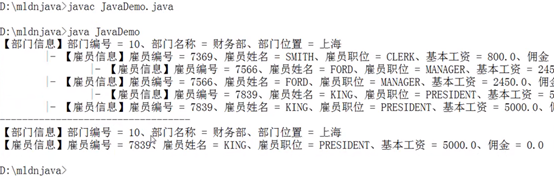364 0945 01204 0699 0《存储漫谈Ceph原理与实践》第三章接入层3.2对象存储RGW
21 0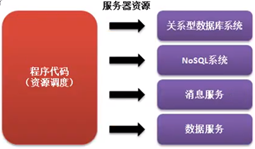564 0《存储漫谈Ceph原理与实践》第三章接入层3.2对象存储RGW（一）
《存储漫谈Ceph原理与实践》第三章接入层3.2对象存储RGW
29 006.Java网络编程（java GUI实现的聊天界面）

1139 0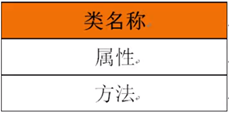592 0920 0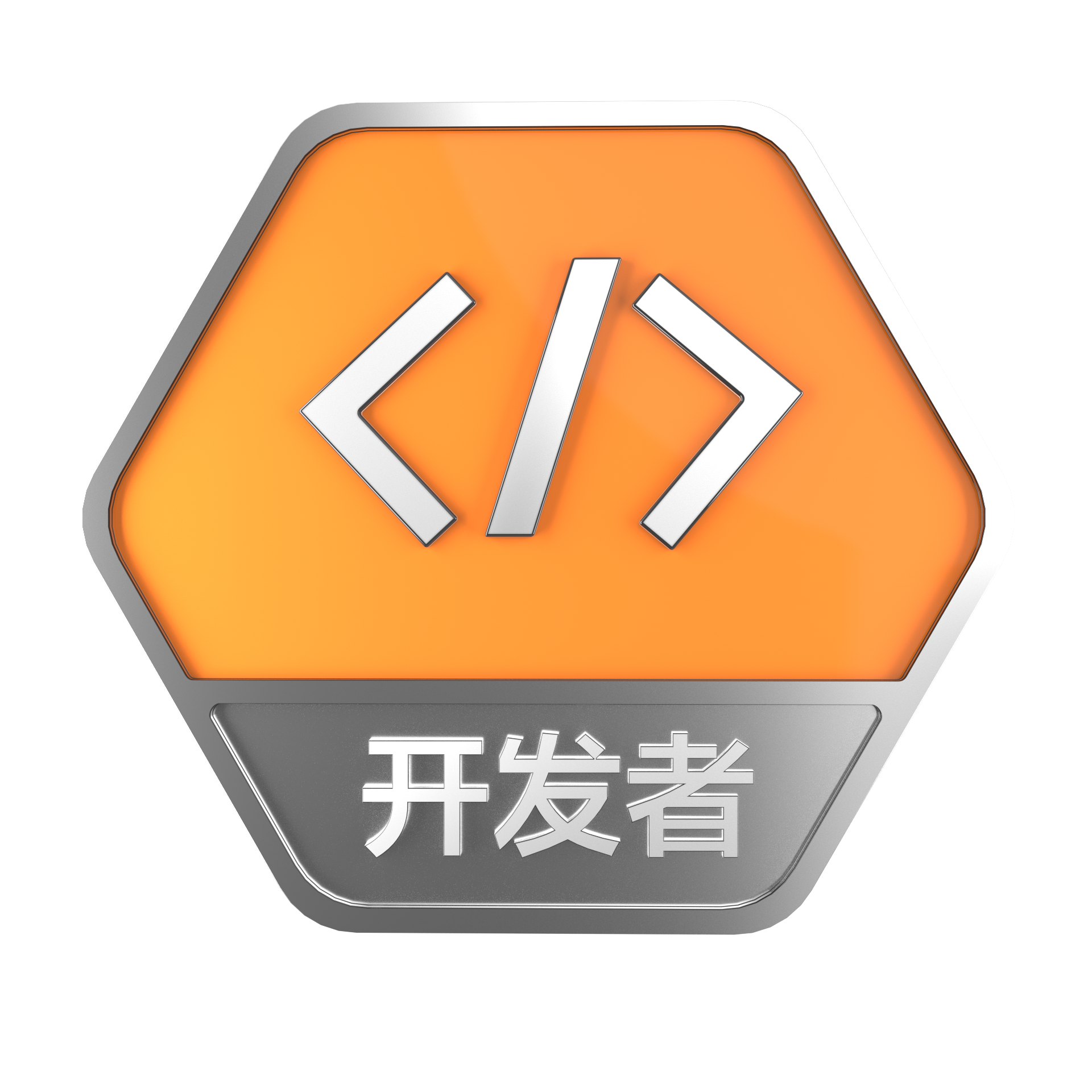+关注
1172

2

Java开发者成长课堂，课程资料学习，实战案例解析，Java工程师必备词汇等你来~
+ 订阅

《2021云上架构与运维峰会演讲合集》

《零基础CSS入门教程》

《零基础HTML入门教程》